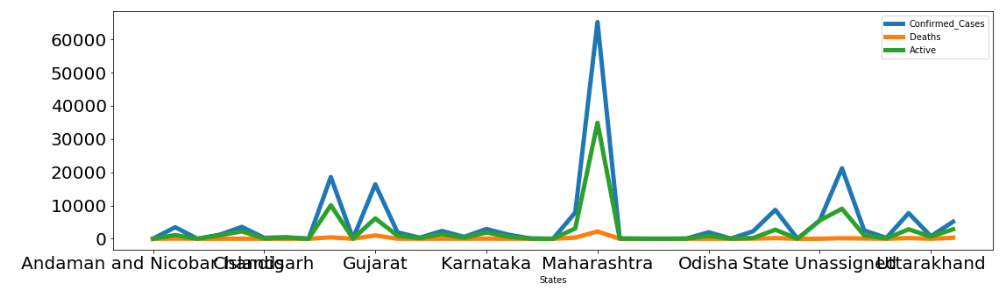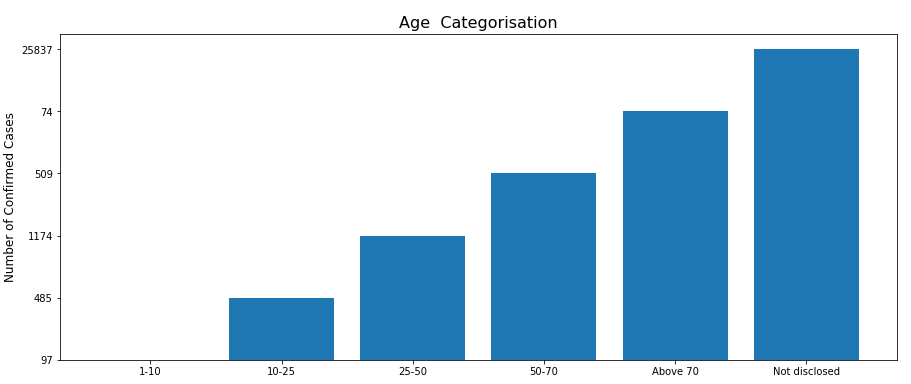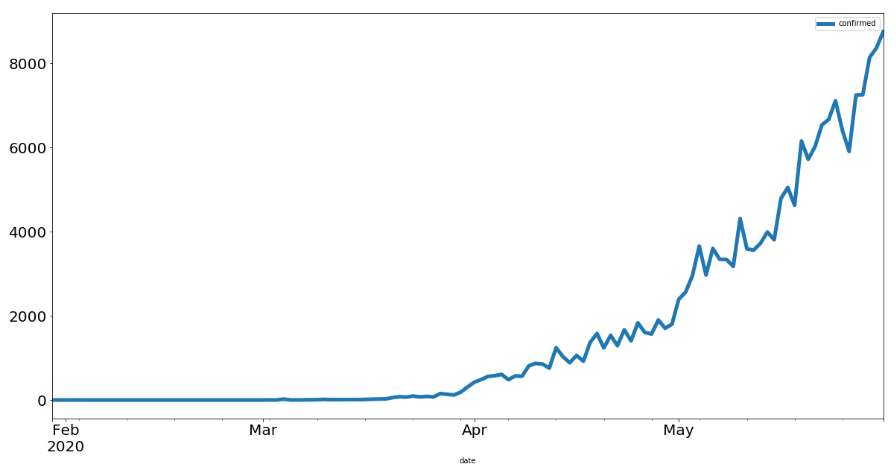# COVID-19 in India

`import requestsimport pandas as pdimport numpy as npimport matplotlib.pyplot as plt`
`response = requests.get("https://api.covid19india.org/data.json")data1 = response.json()response1 = requests.get("https://thecampfire.in/api/daily_state_count")data2 = response1.json()`
`state_list = []for i in range (0, len(data2)-1):    state_names = (data2[i])    state_confirmed = (int(data2[i]['confirmed']))    state_deaths = (int(data2[i]['deaths']))    state_active = (int(data2[i]['active']))    state_list.append([state_names,state_confirmed,state_deaths,state_active])state_data = pd.DataFrame(state_list,              columns=['States','Confirmed_Cases', 'Deaths','Active'])`
`state_data.head()`
`state_data.tail()`
`state_data_plot = pd.DataFrame(state_data.iloc[:,0:].values, columns = ['States','Confirmed_Cases', 'Deaths','Active'])state_data_plot.set_index('States', inplace=True)state_data_plot.plot(figsize=(18,5), linewidth=5, fontsize=20)`Graph showing the pattern of COVID-19 in different states of India
`covid_data_states = state_data.sort_values(by='Confirmed_Cases', ascending=False)fig=plt.figure(figsize=(15, 6))plt.bar(covid_data_states['States'][:5],covid_data_states['Active'][:5],align='center')plt.ylabel('Number of Confirmed Cases', size = 12)plt.title('States with maximum confirmed cases', size = 16)`
`response3= requests.get("https://thecampfire.in/api/get_daily_count")data4 = response3.json()print("Current active cases in India as of {} ----> {} ".format(data4['lastupdatedtime'], data4['active']))print("Current confirmed cases in India as of {} ----> {} ".format(data4['lastupdatedtime'], data4['confirmed']))print("Current death cases in India as of {} ----> {} ".format(data4['lastupdatedtime'], data4['deaths']))`
`response2 = requests.get("https://thecampfire.in/api/state_data")data3 = response2.json()#state = print("Enter state name to get all district details: ")state = "West Bengal"for i in range (0, len(data3)):    if data3[i]['state'] == state:        selected_state_districts = data3[i]['districtData']        for i in selected_state_districts:            print(i['district'])            print("Confirmed: ",i['confirmed'])            print("Active: ",i['active'])            print("Deceased: ",i['deceased'])            print('------------------------')`
`response4 = requests.get("https://thecampfire.in/api/age_count_data")data5 = response4.json()age_list = pd.DataFrame(data5.items())age_cat = ["1-10","10-25","25-50","50-70","Above 70","Not disclosed"]fig=plt.figure(figsize=(15, 6))plt.bar(age_cat,age_list,align='center')plt.ylabel('Number of Confirmed Cases', size = 12)plt.title('Age  Categorisation', size = 16)`Bar graph displaying the age categorisation for the confirmed COVID cases in India.
`response5 = requests.get("https://thecampfire.in/api/daily_total_updates")data6 = response5.json()covid_updates = pd.DataFrame(data6, columns=['Date','Confirmed','Recovered','Deceased'])`
`covid_updates.head()`
`covid_updates.tail()`
`response = requests.get("https://api.covid19india.org/data.json")data1 = response.json()case_time_series_data = data1['cases_time_series']data_len = len(case_time_series_data)data = []#totalDataList = []for i in range (0, data_len):    #print(i)    date = case_time_series_data[i]['date'] + "2020"    #print(date)    confirmed = int(case_time_series_data[i]['dailyconfirmed'])    deceased = int(case_time_series_data[i]['dailydeceased'])    recovered = int(case_time_series_data[i]['dailyrecovered'])    totalconfirmed = int(case_time_series_data[i]['totalconfirmed'])    data.append([date,confirmed,deceased,recovered,totalconfirmed])`
`plot_data = pd.DataFrame(df.iloc[:,0:].values, columns = ['date','confirmed','deceased','recovered','totalconfirmed'])`
`plot_data[['confirmed']].tail()`

# Check Stationarity of the Time-Series

`plot_data[['confirmed']].plot(figsize=(20,10), linewidth=5, fontsize=20)`The trend of the confirmed number of COVID patients in India increasing on a daily basis
`confirmed = plot_data[['confirmed']]`
`from statsmodels.tsa.stattools import adfullerdef check_time_series_stationarity(timeseries):    rolmean = pd.Series(timeseries).rolling(window=15).mean()    rolstd = pd.Series(timeseries).rolling(window=15).std()    orig = plt.plot(timeseries, color='blue',label='Original')    mean = plt.plot(rolmean, color='red', label='Rolling Mean')    std = plt.plot(rolstd, color='black', label = 'Rolling Std')    plt.legend(loc='best')    plt.title('Rolling Mean & Standard Deviation')    plt.show(block=False)        print('------------------------------')    print('Dickey-Fuller Test')    dftest = adfuller(timeseries, autolag='AIC')    dfoutput = pd.Series(dftest[0:4], index=['Test Statistic','p-value','#Lags Used','Number of Observations Used'])    for key,value in dftest.items():        dfoutput['Critical Value (%s)'%key] = value    print(dfoutput)`
`check_time_series_stationarity(confirmed)`

# Process of Estimating & Eliminating Trend from Time-Series

`#Estimating & Eliminating Trendconfirmed_log = np.log(confirmed)plt.plot(confirmed_log)`
`#Moving averagemoving_avg = pd.Series(confirmed_log).rolling(window=15).mean()plt.plot(confirmed_log)plt.plot(moving_avg, color='red')`
`confirmed_log_moving_avg_diff = confirmed_log - moving_avgconfirmed_log_moving_avg_diff.head(15)`
`confirmed_log_moving_avg_diff.dropna(inplace=True)check_time_series_stationarity(confirmed_log_moving_avg_diff)`
`from statsmodels.tsa.seasonal import seasonal_decomposecnf = plot_data['confirmed']for i in range (0, len(cnf)):    val = cnf[i]    if val == 0:        cnf[i] = 1decomposition = seasonal_decompose(cnf)trend = decomposition.trendseasonal = decomposition.seasonalresidual = decomposition.residfig=plt.figure(figsize=(15, 6))plt.subplot(411)plt.plot(cnf, label='Original')plt.legend(loc='best')plt.subplot(412)plt.plot(trend, label='Trend')plt.legend(loc='best')plt.subplot(413)plt.plot(seasonal,label='Seasonality')plt.legend(loc='best')plt.subplot(414)plt.plot(residual, label='Residuals')plt.legend(loc='best')plt.tight_layout()`
`cnf_decompose = residualcnf_decompose.dropna(inplace=True)check_time_series_stationarity(cnf_decompose)`